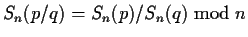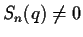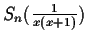Next: Field/congruence enlargement Up: Modular mappings Previous: Modular mappings

## Rational expressions

If a division by 0 occurs, i.e.and Sn(q)=0, then we try to prove (recursively) that q is identically zero. If it is true, then the original expression does not make mathematical sense. If we have proof that the denominator is not zero, this means that we have found an n for which, and hence can continue computing the signature of the original expression with this n. If the division fails because Sn(q) | n, when n is not prime, then we can try with different values of n. In some cases, when there are other restrictions on n, it may not be possible to compute the division. For example, if n is restricted to be a multiple of 2, then the expressioncannot be computed.

Gaston Gonnet
1999-07-04